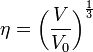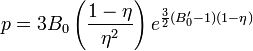# Rose-Vinet (Universal) equation of state

## Vinet

In order to rectify the excessive stiffness of the Murnaghan equation of state as well as represent the exponential dependence of the repulsion as solid undergoes strong compression, Vinet proposed an equation of state, known as either the Vinet equation of state or Universal equation of state. The equation of state was formulated so that one form could represent all solids over a reasonably wide range of pressures, depending only on data for the calibration point. Using the shorthand for the cube root specific volume:$\eta=\left(\frac{V}{V_0}\right)^{\frac{1}{3}}$

the equation of state is (Eq. 4.1):$p=3B_0\left(\frac{1-\eta}{\eta^2}\right)e^{\frac{3}{2}(B_0'-1)(1-\eta)}$

Note: there is a possibility that this equation of state was originally proposed in 1981 by Stacey et al. .